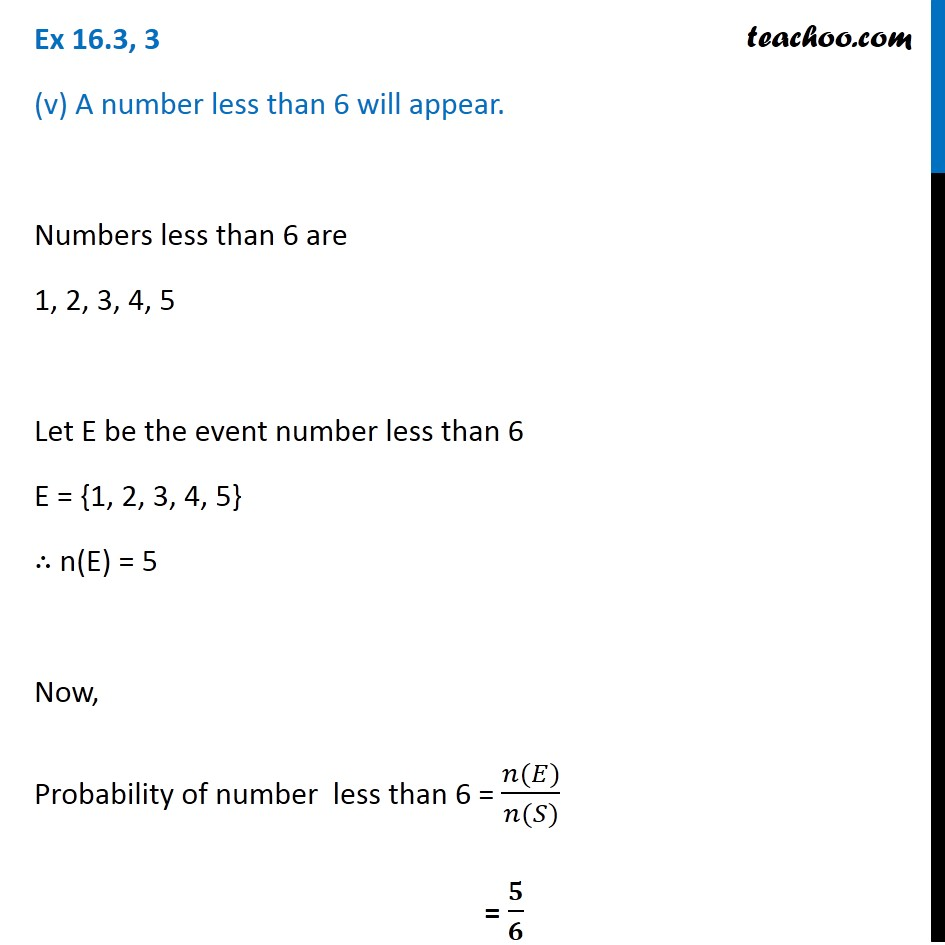Ex 14.2

Chapter 14 Class 11 Probability
Serial order wiseLearn in your speed, with individual attention - Teachoo Maths 1-on-1 Class

### Transcript

Ex 14.2, 3 (v) A number less than 6 will appear. Numbers less than 6 are 1, 2, 3, 4, 5 Let E be the event number less than 6 E = {1, 2, 3, 4, 5} ∴ n(E) = 5 Now, Probability of number less than 6 = (𝑛(𝐸))/(𝑛(𝑆)) = 𝟓/𝟔